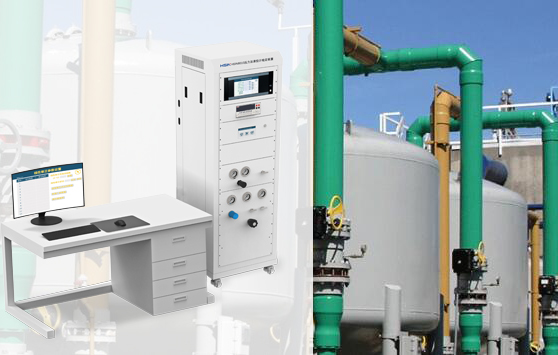### 常见问答### 常见问答h = p /（ρ * g）

p = 静水压力 [巴（表压）]

ρ = 液体的比重 [kg/m³]

g = 重力或重力加速度 [m/s²]

h = 液柱高度 [m] = 液位

h = （p2 - p1） / （（ρ * g）

p2 = 静水压力 [bar]

p1= 容器中封闭气体的压力 [bar]

ρ = 流体的比重 [kg/m³]

g = 重力或重力加速度 [m/s²]

h = 液柱高度 [m] = 液位

### 推荐新闻

010-56674468
•